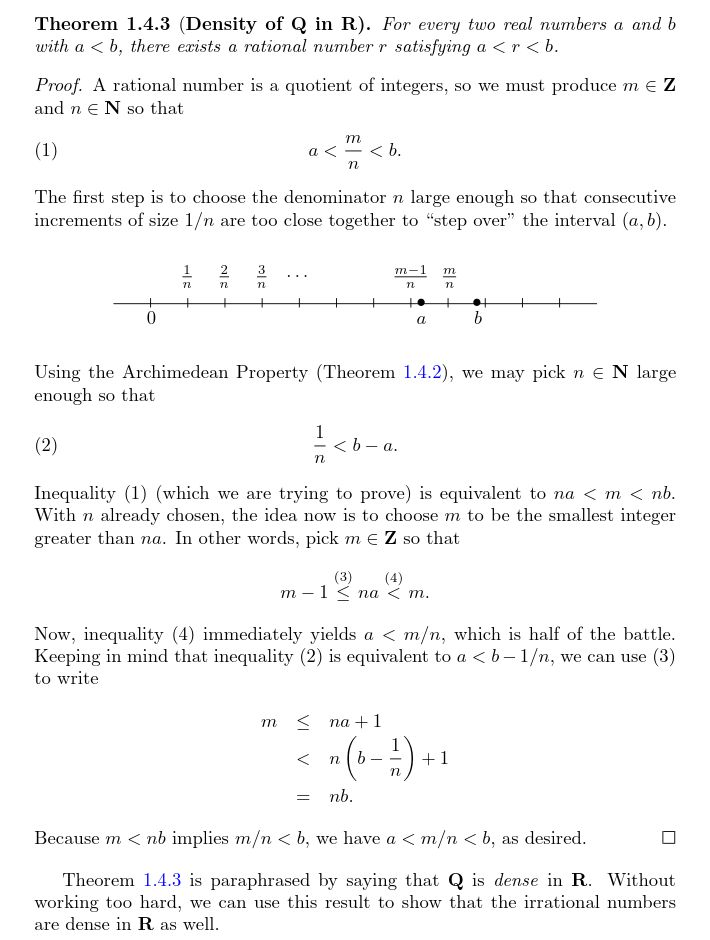Show Question
Math and science::Analysis::Tao::05. The real numbers

# Archimedean property

The Archimedean property is a property of the reals. Roughly, it says: there are no infinitely small or infinitely large elements, when compared to the rationals. It also says that the naturals are not bounded by any real.

### Archimedean property

1. Given any number $$x \in \mathbb{R}$$, there exists an $$n \in \mathbb{N}$$ satisfying $$n > x$$.
2. Given any real number $$x > 0$$, there exists an $$n \in \mathbb{N}$$ satisfying $$\frac{1}{n} < x$$.

This is Abbott's description of the Archimedean property.

Tao presents the more common form which goes like:

For any two positive reals $$x$$ and $$y$$ there exists a natural number $$n$$ such that $$ny > x$$.

### Density of $$\mathbb{Q}$$ in $$\mathbb{R}$$

The Archimedean property leads to an important result:

For any reals $$a, b \in \mathbb{R}$$ such that $$a < b$$ there exists a rational $$q \in \mathbb{Q}$$ such that $$a < q < b$$.

### Proof

The below proof assumes the truth of two statements:

1. Interspersing of integers by rationals: let $$x$$ be a rational number, then there exits an integer $$n$$ such that $$n \ge x \ge n+1$$.
2. Cauchy sequences (sequences of rationals) are bounded (by rationals). Note that a sequence $$(a_n)_{n=1}^{\infty}$$ is bounded by $$M \in \mathbb{Q}$$ iff $$\forall i \in \mathbb{N}, |a_i| \le M$$.

Proof.

• First prove 1. Let $$x \in \mathbb{R}$$. $$x$$ is a Cauchy sequence of rationals, and is thus bounded by rationals. Any rational is also bounded by integers, so we have the desired result by transitivity.
• For part 2, rewrite as $$n > \frac{1}{y}$$ and apply part 1.

### Density of $$\mathbb{Q}$$ in $$\mathbb{R}$$, proof.Tao, p114, p115
Abbott, p21